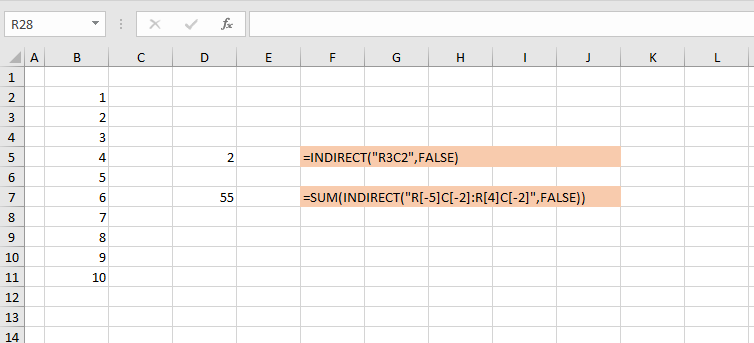# Direct vs indirect referencing

## The INDIRECT function

The Excel INDIRECT function returns a reference specified by a text string.

SYNTAX: INDIRECT(ref_text, [a1])

Figure 1 shows examples of the INDIRECT function (two stage), and a direct reference (one stage). The custom GetCF function is used to show the cell formula.Fig 1: Direct reference and Indirect reference – cell D5 contains a direct reference, cell D11 contains an indirect reference via cell F7 that points to cell B8
• Cell D5: =B2, this is a direct, one stage reference to B2, and the formula returns the value 1
• Cell D11: =INDIRECT(F7), where cell F7 contains the text string B8. Without the INDIRECT function, D11 would display the text string B8, but the INDIRECT function points to cell B8 and returns the value 7

## When to use the INDIRECT function

The INDIRECT function is often used in conjunction with the concatenation operator (&) to construct references from labels. For example =VLOOKUP($A14,INDIRECT(B$13&".ax!"&B\$13),8,FALSE) from the link at xlf Vlookup w/ indirect

## INDIRECT allows R1C1 reference style in A1 workbook

Using the INDIRECT function is one way of using R1C1 reference style in an A1 workbook, or A1 reference style in an R1C1 workbook.Fig 2: INDIRECT function – with A1 argument set to False. This allows R1C1 style in the A1 workbook